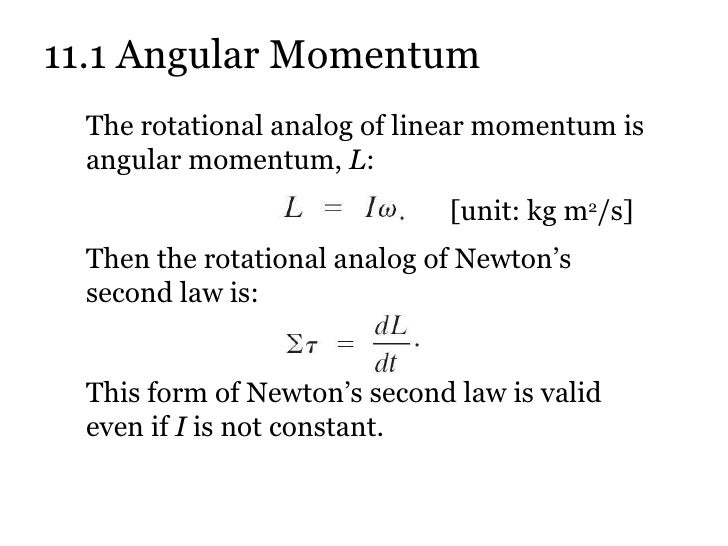# Question 5a15b

Dec 28, 2014

Answer : The SI unit for angular momentum is $\setminus \quad k g . {m}^{2.} {s}^{- 1}$ and its dimension is $M . {L}^{2.} {T}^{- 1}$.

Explanation : Angular Momentum is the rotational motion equivalent of the Linear Momentum of Translational motion. It is defined as the moment of Linear Momentum.

$\setminus \vec{L} \setminus \equiv \setminus \vec{r} \setminus \times \setminus \vec{p} = \setminus \vec{r} \setminus \times m \setminus \vec{v}$

L = r.mv.\sin\theta; \qquad \sin\theta # is a unitless quantity.
SI units for $m , r$ and $v$ are, respectively, $k g$, $m$ and $m . {s}^{- 1}$.